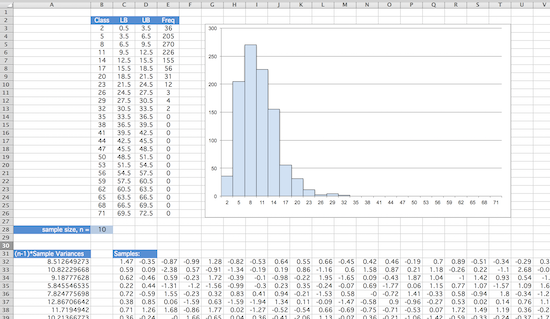## Excel Project: The Chi Square Distribution

When samples of size $n$ are taken from a population that follows a standard normal distribution, and the sample variances $s^2$ are found for each sample, the distribution of values of the form $\chi^2 = (n-1)s^2$ follows what is called a chi-square distribution.

Simulate this distribution in Excel, by doing the following:

1. First create a 1000 row by 30 column block of data that follows a standard normal distribution. The NORM.INV() function may be helpful in this regard. We will use this block of data to create random samples of various sizes.

2. Establish in some cell (such as B28 in the image below) a place where one can enter the desired sample size (max 30). Suppose the value entered in this cell is $n$. Treat the first $n$ values in each row of your data block as a sample and find $\chi^2 = (n-1)s^2$ for the resulting 1000 samples. You may find the OFFSET() function in Excel useful to this end.

3. Create a histogram of these $\chi^2$ values so created. Use classes of width 3, centered at 2, 5, 8, ... to 71.

4. Experiment with different sample sizes (up to a maximum of 30), by entering different values in the appropriate cell (again, like B28 below). Describe the resulting distributions seen. Comment in particular on their shape, center, and spread.